Trending Today#### Caverton 2019H1: Higher Valuation On Improved Earnings, Yield, Lower CostsCompany: Caverton Offshore Support Group Plc
Current Market Price: N2.30
Year High: N3.26
Year Low: N1.90
Fair Value: N6.66
Equity Analyst: Tunde Segun Jeariogbe

Key Investment Ratios
• In this report, we observed the 2019 half-year financial records of Caverton Offsshore Support Group, compared same with that released in the similar result of 2018 to establish growth.
• Performance boosters for the period include Improved the revenue figure, Other Operating Income and a reduced Finance Cost, which took care of the difference in Operating Expenses and finally resulted in an improved Profit at the end of the quarter.
• Up from the three months intrinsic value, we have further stepped up our worth for each unit of Caverton Shares to N6.66, following which have rated it a ‘BUY.’
• The above position is premised on the fact that a large difference exists between the estimated fair value and the current market price of Caverton shares on the floor of the exchange as at the time we compiled this report.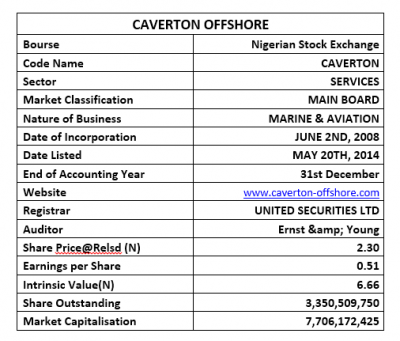Company figures
• The Turnover figure reported for the quarter stood above the comparable period’s by 18.86%, growing from the previously reported N14.22 billion to N16.90 billion.
• Direct Cost equally grew by 15.27% to N10.89 billion, as against the N9.45 billion reported at the end of 2018 half-year.
• Thus, Operating Expenses is currently valued at N6.00 billion, same as 25.98% above the N4.76 billion stated in its 2018 half-year financial performance figures.
• Operating Expenses through the period was valued at N2.77 billion, which is 39.19% above the N1.99 billion spent in 2018 half year.
• Finance Cost on the other hand adjusted down by 14.17% to N1.08 billion from the previously reported N1.26 billion.
• Thus, Profit before Tax reported for the half-year stood at N2.62 billion, a 68.68% improvement over the N1.55 billion earned in 2018.
• Having accounted for Tax Expenses, a total of N1.71 billion was earned at the end of the period, compared to the N962.45 million earned at the end of the first 6 months of 2018.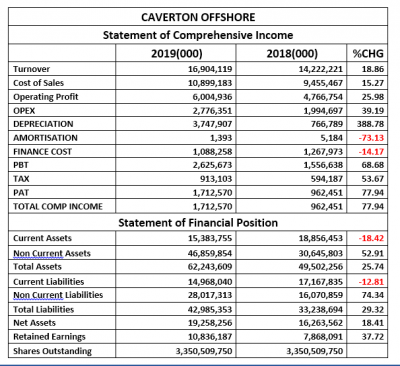• Current Assets of Caverton at the end of the first six months of 2019 was valued at N15.38 billion below the N18.85 billion in 2018 half year.
• Non-Current Assets on the other hand improved by 52.91% to N46.85 billion, as against the previous N30.64 billion valued in its 2018 half-year financials.
• In all, Total Assets grew by 25.74% to stand at N62.24 billion versus N49.50 billion in 2018.
• Current Liabilities, on the other hand, dipped by 12.81% from the previous N17.16 billion to the current estimated value of N14.96 billion.
• Conversely, Non-Current Liabilities grew by 74.34% over the comparable period’s value. The figure moved to N28.01 billion against N16.07 billion.
• Thus, Total Liability is currently valued at N42.98 billion, same as 29.32% above the previously reported N33.23 billion in 2018.
• On the strength of the above, Net Assets was valued at N19.25 billion, up from the 16.26 billion in 2018 half-year earnings.

Financial Strength/Solvency Ratios
• Debt Ratio is currently estimated at 0.69x, 2.85% above the 0.67x estimated in the similar period of 2018. This implies that Total Liabilities is same as 69% of Total Assets.
• Total Debt to Equity was equally estimated at 2.23x as against 2.04x estimated in the comparable half-year. This simply implies that Debt is far higher than the equity through the period.
• It was established that Equity is the same as 31% of Caverton’s Assets Value, which is a 5.83% drop, when compared to the 33% achieved in the similar period of 2018.
• Going by the beta value of 0.37, which is far below unity, we can safely conclude that shares of Caverton is relatively not liquid.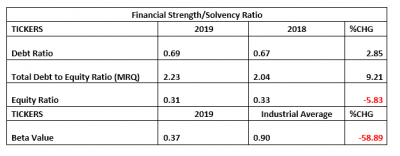Profitability Ratios
• Profit before Tax had been estimated to be 15.53% of the Turnover figure, as against the 10.95% achieved at the end of 2018 half-year.
• The tax rate within the two periods compared is relatively stable with a percentage difference of 13.64%.
• Cost of Sales is 64.48% of Turnover, which is only 3.02% below the 66.48% estimated in 2018.
• Return on Equity stood at 8.89% against 5.92% of 2018.Efficiency Ratios
• Operating Expenses to Turnover value is same as 16.42%, this is 17.10% efficiency improvement from the 14.03% estimated from 2018 half-year financial indices
• Turnover to Total Assets Ratios stood at 27.16% as against 28.73% when this is compared to ratio estimate in the 2018 result, it is a 5.47% drop inefficiency.
• Working Capital Turnover, a ratio that indicates a company’s effectiveness in using its working capital was estimated at 40.66x as against the 8.42x estimated in the similar period of 2018.
• We also tested the Working Capital Ratio, which confirmed that Current Assets stood below the current liabilities at the end of the period, same as in 2018 half-year result.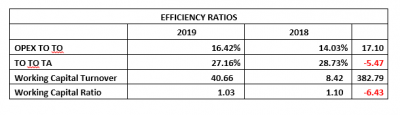Investment/Valuation Ratios
• At the end of the period, the earnings per unit of Caverton is estimated at N0.51, this is 77.94% above the N0.29 earned in 2018 half year.
• P/E-Ratio reduced to 4.05x from the previously estimated 7.28x, please note that the quality of this ratio is established in the fact that there is only a little difference between the share price on the floor of the exchange in the two periods compared
• The said earnings are the same as 22.22% yield of the current market price of Caverton, this is 61.68% yield improvement when compared to the 13.74% yield estimated in 2018.
• Caverton’s Book value is currently estimated at N5.75 against N4.85 in 2018, this is the first confirmation of an undervalued state of its shares on the floor of the exchange.
• Further confirming the undervalued status is the Price to Book value ratio that stood below unity (both periods compared).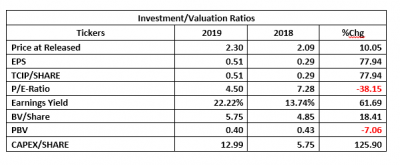Valuation
Boosting factors while attempting to fairly value each unit of Caverton include the improved earnings performance, growing investors’ reward (cash dividend) within 2018 and 2017 financial year. We assumed a reduced growth rate in cash dividend over the past three years and thus, valued each unit of Caverton’s share for N6.66 and Rated it a Buy.

## Market Update